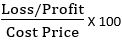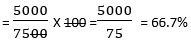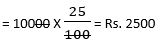## LetsPlayMaths.Com

WELCOME TO THE WORLD OF MATHEMATICS

# Class 5 Profit and Loss

Profit and Loss

Profit / Loss Percentage

Find S.P When C.P and Percentage of Profit / Loss is Known

Find C.P When S.P and Percentageof Profit / Loss are Known

Profit / Loss Test

Profit / Loss Worksheet

## Profit and Loss

A store owner purchases a product for Rs. 785 and sells it for Rs. 880. He got Rs. 95 as gain, which is known as profit.

Profit = Selling Price – Cost Price

Going forward, we will use S.P as selling price and C.P as cost price.

At times, the same store owner must sell his old products at a lesser price than the Cost price. For
example, he purchased a product for Rs. 700 and sold it at Rs. 625. He incurred a loss of Rs. 75.

Loss = Cost Price – Selling Price

If, C.P > S.P, then Loss = C.P – S.P

If, S.P > C.P, then Profit = S.P – C.P

## Profit / Loss Percentage

Most of the time we intend to know the amount of profit or loss a business is making. To find out the percentage of loss or profit we must follow below mentioned steps.

1. Find out the loss/profit
2.Example 1. Bob bought a toy for Rs. 200 and sold it for Rs. 280. Find out his percentage of gain.

Solution. C.P of the toy = Rs. 200

S.P of the toy = Rs. 280

Profit = S.P – C.P = Rs. 280 – Rs. 200 = Rs. 80

Percentage of profitSo, the amount of profit Bob made is 40%.

Example 2. John bought a cycle for Rs. 7500 and he sold it for Rs. 2500 after using it for 4 years. Find out the percentage of loss he incurred.

Solution. C.P of the cycle = Rs. 7500

S.P of the cycle = Rs. 2500

Loss incurred = C.P – S.P = Rs. 7500 – Rs. 2500 = Rs. 5000

Percentage of lossSo, the percentage of loss incurred by John is 66.7%

## Find S.P When C.P and Percentage of Profit / Loss is Known

To find out selling price when cost price and profit/loss are known, we must follow below mentioned steps.

1. Amount of profit/loss = (C.P X Percentage of profit)/loss

2. Selling Price = C.P + Amount of Profit

Selling Price = C.P – Amount of Loss

Example 1. A shopkeeper bought a television for Rs. 10000 and he sold it at profit of 25%. What was the selling price of the television?

Solution. C.P of the television = Rs. 10000

Profit = 25%

Amount of profit = Rs. 10000 X 25%S.P = C.P + Profit Amount

= Rs. 10000 + Rs. 2500 = Rs. 12500

So, the selling price of the television is Rs. 12500.

Example 2. A man bought a car for \$ 25000 and he used it for ten years. If he sold the car at a loss of 35%, then what is the selling price of the car?

Solution. C.P of the car = \$ 25000

Loss = 35%

Amount of loss = \$ 25000 X 35%S.P = C.P - Loss amount = \$ 25000 - \$ 8750 = \$ 16250

So, the selling price of the car is \$ 16250.

## Find C.P When S.P and Percentageof Profit / Loss are Known

To find out cost price when selling price and percentage profit/loss are known, we must follow below mentioned procedures.

C.P + (C.P X Percentage of Profit) = S.P

C.P - (C.P X Percentage of Profit) = S.P

Example 1. A store sold a shirt for a price Rs. 1500 at a profit of 20%. Find out the cost price of the shirt.

Solution. Let's assume C.P of the shirt is ‘Y’

Y + (Y x 20%) = 1500So, the cost price of the shirt is Rs. 1250.

Example 2. During a festival time, a dealer sold lots of bike at Rs. 44000 per piece. If the dealer incurred 12% loss per bike, then what is the cost price per bike?

Solution. Say C.P of the bike is ‘A’

A – A X 12% = 44000So, the cost price of the bike is Rs. 50000.

## Class-5 Profit and Loss Worksheet

Profit & Loss Test - 1

Profit & Loss Test - 2

## Class-5 Profit and Loss Worksheet

Profit & Loss Worksheet - 1

Profit & Loss Worksheet - 2

Profit & Loss Worksheet - 3